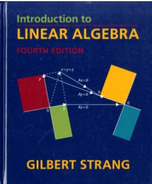Introduction To Linear Algebra - 4 Edition - Chapter 2.7 - Problem 22
Register Now

Join StudySoup

Get Full Access to Introduction To Linear Algebra - 4 Edition - Chapter 2.7 - Problem 229780980232714

# Solved: Questions 22-24 are aboutthe factorizations PA = L U and A = LIP 1 U 1-

Introduction to Linear Algebra | 4th Edition

Problem 22

Questions 22-24 are aboutthe factorizations PA = L U and A = LIP 1 U 1-

Accepted Solution
Step-by-Step Solution:
Step 1 of 3

M303 Section 5.3 Notes- Diagonalization 12-7-16  Recall diagonal matrix- only (possible) nonzero entries are on diagonal o Ex. Simple to compute powers 5 0 2 5 0 5 0 52 0  [0 3] = [0 3 0 3 ]= [ 2] 3 2 02 3 3  [5 0] = [5 0 ] [5 0]= [5 0 ][5 0]= [5 0 ] 0 3 0 3 0 3 0 3 2 0 3 0 33 5 0 5 0  [0 3] = [0 3] o Commute in multiplication  Not all matrices can be diagonal, but class of diagonalizable matrices exists that has many of same properties of diagonal matrices o × matrix diagonali

###### Chapter 2.7, Problem 22 is Solved

Step 2 of 3

Step 3 of 3

Unlock Textbook Solution